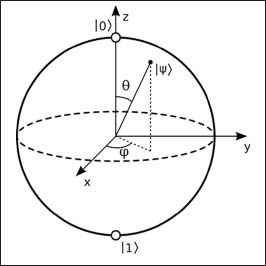NextPrevious

# What is mathematical physics?

Mathematical physics uses the concepts of statistical mechanics and quantum field theory. But it is not the same as theoretical physics. Mathematical physics studies physics on a more abstract and meticulous level. Theoretical physics entails less mathematics than mathematical physics and has more to do with experimental physics. But like many fields of science, defining mathematical physics is not easy. For example, still another definition of modern mathematical physics states that it entails all areas of mathematics other than classical mathematical physics.A diagram of a bloch sphere, or diagram of a qubit, which is the basic building block of quantum computers. Named after Swiss physicist Felix Bloch (1905–1983), the bloch sphere has two possible states, which can represent the 0s and 1s used in computing. It took highly advanced mathematics to develop this concept!

Close

This is a web preview of the "The Handy Math Answer Book" app. Many features only work on your mobile device. If you like what you see, we hope you will consider buying. Get the App